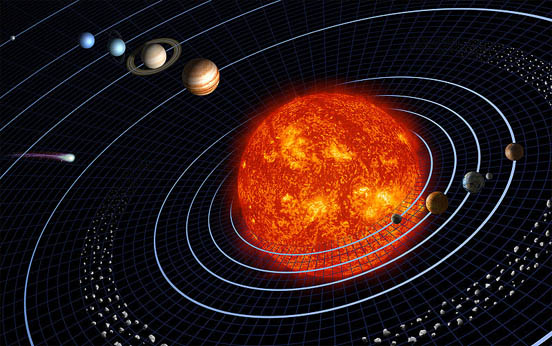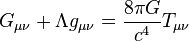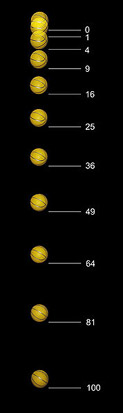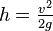GravitationBy Wikipedia,
the free encyclopedia,

http://en.wikipedia.org/wiki/GravityGravitation keeps the planets in orbit about the Sun. (Not to scale)

Gravitation is a natural phenomenon by which objects with mass attract one another. In everyday life, gravitation is most commonly thought of as the agency which lends weight to objects with mass. Gravitation compels dispersed matter to coalesce, thus it accounts for the very existence of the Earth, the Sun, and most of the macroscopic objects in the universe. It is responsible for keeping the Earth and the other planets in their orbits around the Sun; for keeping the Moon in its orbit around the Earth, for the formation of tides; for convection (by which fluid flow occurs under the influence of a temperature gradient and gravity); for heating the interiors of forming stars and planets to very high temperatures; and for various other phenomena that we observe. Modern physics describes gravitation using the general theory of relativity, in which gravitation is a consequence of the curvature of spacetime which governs the motion of inertial objects. The simpler Newton's law of universal gravitation provides an excellent approximation for most calculations.

The terms gravitation and gravity are mostly interchangeable in everyday use, but a distinction is made in scientific usage. "Gravitation" is a general term describing the phenomenon by which bodies with mass are attracted to one another, while "gravity" refers specifically to the net force exerted by the Earth on objects in its vicinity as well as by other factors, such as the Earth's rotation.

## History of gravitational theory

### Scientific revolution

Modern work on gravitational theory began with the work of Galileo Galilei in the late 16th and early 17th centuries. In his famous (though possibly apocryphal) experiment dropping balls from the Tower of Pisa, and later with careful measurements of balls rolling down inclines, Galileo showed that gravitation accelerates all objects at the same rate. This was a major departure from Aristotle's belief that heavier objects are accelerated faster. Galileo correctly postulated air resistance as the reason that lighter objects may fall more slowly in an atmosphere. Galileo's work set the stage for the formulation of Newton's theory of gravity.

### Newton's theory of gravitation

In 1687, English mathematician Sir Isaac Newton published Principia, which hypothesizes the inverse-square law of universal gravitation. In his own words, “I deduced that the forces which keep the planets in their orbs must [be] reciprocally as the squares of their distances from the centers about which they revolve: and thereby compared the force requisite to keep the Moon in her Orb with the force of gravity at the surface of the Earth; and found them answer pretty nearly.”

Newton's theory enjoyed its greatest success when it was used to predict the existence of Neptune based on motions of Uranus that could not be accounted by the actions of the other planets. Calculations by John Couch Adams and Urbain Le Verrier both predicted the general position of the planet, and Le Verrier's calculations are what led Johann Gottfried Galle to the discovery of Neptune.

Ironically, it was another discrepancy in a planet's orbit that helped to point out flaws in Newton's theory. By the end of the 19th century, it was known that the orbit of Mercury showed slight perturbations that could not be accounted for entirely under Newton's theory, but all searches for another perturbing body (such as a planet orbiting the Sun even closer than Mercury) had been fruitless. The issue was resolved in 1915 by Albert Einstein's new General Theory of Relativity, which accounted for the small discrepancy in Mercury's orbit.

Although Newton's theory has been superseded, most modern non-relativistic gravitational calculations are still made using Newton's theory because it is a much simpler theory to work with than General Relativity, and gives sufficiently accurate results for most applications.

### Gravitational torsion, weak equivalence principle and gravitational gradient

Loránd Eötvös published on surface tension between 1876 and 1886. The Torsion or Eötvös balance, designed by Hungarian Baron Loránd Eötvös, is a sensitive instrument for measuring the density of underlying rock strata. The device measures not only the direction of force of gravity, but the change in the force of gravity's extent in horizontal plane. It determines the distribution of masses in the Earth's crust. The Eötvös torsion balance, an important instrument of geodesy and geophysics throughout the whole world, studies the Earth's physical properties. It is used for mine exploration, and also in the search for minerals, such as oil, coal and ores. Eötvös' law of capillarity (weak equivalence principle) served as a basis for Einstein's theory of relativity. (Capillarity: the property or exertion of capillary attraction of repulsion, a force that is the resultant of adhesion, cohesion, and surface tension in liquids which are in contact with solids, causing the liquid surface to rise - or be depressed...) These experiments demonstrate that all objects fall at the same rate with negligible friction (including air resistance). The simplest way to test the weak equivalence principle is to drop two objects of different masses or compositions in a vacuum, and see if they hit the ground at the same time. More sophisticated tests use a torsion balance of a type invented by Loránd Eötvös. Satellite experiments are planned for more accurate experiments in space. They verify the weak principle.

### General relativity

Fundamental concepts General relativity$G_{\mu \nu} + \Lambda g_{\mu \nu}= {8\pi G\over c^4} T_{\mu \nu}\,$ Einstein field equations Introduction to... Mathematical formulation of... Resources Special relativity Equivalence principle World line · Riemannian geometry

In general relativity, the effects of gravitation are ascribed to spacetime curvature instead of a force. The starting point for general relativity is the equivalence principle, which equates free fall with inertial motion, and describes free-falling inertial objects as being accelerated relative to non-inertial observers on the ground. In Newtonian physics, however, no such acceleration can occur unless at least one of the objects is being operated on by a force.

Einstein proposed that spacetime is curved by matter, and that free-falling objects are moving along locally straight paths in curved spacetime. These straight lines are called geodesics. Like Newton's First Law, Einstein's theory stated that if there is a force applied to an object, it would deviate from the geodesics in spacetime. For example, we are no longer following the geodesics while standing because the mechanical resistance of the Earth exerts an upward force on us. Thus, we are non-inertial on the ground. This explains why moving along the geodesics in spacetime is considered inertial.

Einstein discovered the field equations of general relativity, which relate the presence of matter and the curvature of spacetime and are named after him. The Einstein field equations are a set of 10 simultaneous, non-linear, differential equations. The solutions of the field equations are the components of the metric tensor of spacetime. A metric tensor describes a geometry of spacetime. The geodesic paths for a spacetime are calculated from the metric tensor.

Notable solutions of the Einstein field equations include:

The tests of general relativity included:

• General relativity accounts for the anomalous perihelion precession of Mercury.
• The prediction that time runs slower at lower potentials has been confirmed by the Pound-Rebka experiment, the Hafele-Keating experiment, and the GPS.
• The prediction of the deflection of light was first confirmed by Arthur Eddington in 1919. The Newtonian corpuscular theory also predicted a lesser deflection of light, but Eddington found that the results of the expedition confirmed the predictions of general relativity over those of the Newtonian theory. However this interpretation of the results was later disputed. More recent tests using radio interferometric measurements of quasars passing behind the Sun have more accurately and consistently confirmed the deflection of light to the degree predicted by general relativity. See also gravitational lensing.
• The time delay of light passing close to a massive object was first identified by Irwin Shapiro in 1964 in interplanetary spacecraft signals.
• Gravitational radiation has been indirectly confirmed through studies of binary pulsars.
• Alexander Friedmann in 1922 found that Einstein equations have non-stationary solutions (even in the presence of the cosmological constant). In 1927 Georges Lemaître showed that static solutions of the Einstein equations, which are possible in the presence of the cosmological constant, are unstable, and therefore the static universe envisioned by Einstein could not exist. Later, in 1931, Einstein himself agreed with the results of Friedmann and Lemaître. Thus general relativity predicted that the Universe had to be non-static—it had to either expand or contract. The expansion of the universe discovered by Edwin Hubble in 1929 confirmed this prediction.

### Gravity and quantum mechanics

Several decades after the discovery of general relativity it was realized that general relativity is incompatible with quantum mechanics. It is possible to describe gravity in the framework of quantum field theory like the other fundamental forces, such that the attractive force of gravity arises due to exchange of virtual gravitons, in the same way as the electromagnetic force arises from exchange of virtual photons. This reproduces general relativity in the classical limit. However, this approach fails at short distances of the order of the Planck length, where a more complete theory of quantum gravity (or a new approach to quantum mechanics) is required. Many believe the complete theory to be string theory, or more currently M Theory.

## Specifics

### Earth's gravity

Every planetary body (including the Earth) is surrounded by its own gravitational field, which exerts an attractive force on all objects. Assuming a spherically symmetrical planet (a reasonable approximation), the strength of this field at any given point is proportional to the planetary body's mass and inversely proportional to the square of the distance from the center of the body.

The strength of the gravitational field is numerically equal to the acceleration of objects under its influence, and its value at the Earth's surface, denoted g, is approximately expressed below as the standard average.

g = 9.8 m/s = 32.2 ft/s

This means that, ignoring air resistance, an object falling freely near the Earth's surface increases its velocity with 9.8 m/s (32.2 ft/s or 22 mph) for each second of its descent. Thus, an object starting from rest will attain a velocity of 9.8 m/s (32.2 ft/s) after one second, 19.6 m/s (64.4 ft/s) after two seconds, and so on, adding 9.8 m/s (32.2 ft/s) to each resulting velocity. Also, again ignoring air resistance, any and all objects, when dropped from the same height, will hit the ground at the same time.

According to Newton's 3rd Law, the Earth itself experiences an equal and opposite force to that acting on the falling object, meaning that the Earth also accelerates towards the object (until the object hits the earth, then the Law of Conservation of Energy states that it will move back with the same acceleration with which it initially moved forward, canceling out the two forces of gravity.). However, because the mass of the Earth is huge, the acceleration of the Earth by this same force is negligible, when measured relative to the system's center of mass.

### Equations for a falling body near the surface of the EarthBall falling freely under gravity. See text for description.

Under an assumption of constant gravity, Newton’s law of gravitation simplifies to F = mg, where m is the mass of the body and g is a constant vector with an average magnitude of 9.81 m/s². The acceleration due to gravity is equal to this g. An initially-stationary object which is allowed to fall freely under gravity drops a distance which is proportional to the square of the elapsed time. The image on the right, spanning half a second, was captured with a stroboscopic flash at 20 flashes per second. During the first 1/20th of a second the ball drops one unit of distance (here, a unit is about 12 mm); by 2/20ths it has dropped at total of 4 units; by 3/20ths, 9 units and so on.

Under the same constant gravity assumptions, the potential energy, Ep, of a body at height h is given by Ep = mgh (or Ep = Wh, with W meaning weight). This expression is valid only over small distances h from the surface of the Earth. Similarly, the expression$h = \tfrac{v^2}{2g}$ for the maximum height reached by a vertically projected body with velocity v is useful for small heights and small initial velocities only.

### Gravity and astronomy

The discovery and application of Newton's law of gravity accounts for the detailed information we have about the planets in our solar system, the mass of the Sun, the distance to stars, quasars and even the theory of dark matter. Although we have not traveled to all the planets nor to the Sun, we know their masses. These masses are obtained by applying the laws of gravity to the measured characteristics of the orbit. In space an object maintains its orbit because of the force of gravity acting upon it. Planets orbit stars, stars orbit galactic centers, galaxies orbit a center of mass in clusters, and clusters orbit in superclusters. The force of gravity is proportional to the mass of an object and inversely proportional to the square of the distance between the objects.

In general relativity, gravitational radiation is generated in situations where the curvature of spacetime is oscillating, such as is the case with co-orbiting objects. The gravitational radiation emitted by the solar system is far too small to measure. However, gravitational radiation has been indirectly observed as an energy loss over time in binary pulsar systems such as PSR 1913+16. It is believed that neutron star mergers and black hole formation may create detectable amounts of gravitational radiation. Gravitational radiation observatories such as LIGO have been created to study the problem. No confirmed detections have been made of this hypothetical radiation, but as the science behind LIGO is refined and as the instruments themselves are endowed with greater sensitivity over the next decade, this may change.

## Anomalies and discrepancies

There are some observations that are not adequately accounted for, which may point to the need for better theories of gravity or perhaps be explained in other ways.

• Pioneer anomaly: The two Pioneer spacecraft seem to be slowing down in a way which has yet to be explained.
• Flyby anomaly: Various spacecraft have experienced greater accelerations during slingshot maneuvers than expected.
• Accelerating expansion: The expansion of the universe seems to be speeding up. Dark energy has been proposed to explain this. A recent alternative explanation is that the geometry of space is not homogeneous (due to clusters of galaxies) and that when the data are reinterpreted to take this into account, the expansion is not speeding up after all, however this conclusion is disputed.
• Anomalous increase of the AU: Recent measurements indicate that planetary orbits are expanding faster than if this was solely through the sun losing mass by radiating energy.
• Extra energetic photons: Photons travelling through galaxy clusters should gain energy and then lose it again on the way out. The accelerating expansion of the universe should stop the photons returning all the energy, but even taking this into account photons from the cosmic background radiation gain twice as much energy as expected. This may indicate that gravity falls off faster than inverse-squared at certain distance scales.
• Dark flow: Surveys of galaxy motions have detected a mystery dark flow towards an unseen mass. Such a large mass is too large to have accumulated since the big bang using current models and may indicate that gravity falls off slower than inverse-squared at certain distance scales.
• Extra massive hydrogen clouds: The spectral lines of the Lyman alpha forest suggest that hydrogen clouds are more clumped together at certain scales than expected and, like dark flow, may indicate that gravity falls off slower than inverse-squared at certain distance scales.

## References

Published in July 2009.